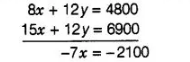# Vijay had some bananas and he divided

Question:

Vijay had some bananas and he divided them into two lots A and B. He sold the first lot at the rate of ₹ 2 for 3 bananas and the second lot at the rate of

₹ 1 per banana and got a total of ₹ 400 If he had sold the first lot at the rate of ₹ 1 per banana and the second lot at the rate of ₹ 4 for 5 bananas, his

total collection would have been ₹ 460. Find the total nmber of bananas he had.

Solution:

Let the number of bananas in lots A and B be x and y, respectively

Case I Cost of the first lot at the rate of ₹ 2 for 3 bananas + Cost of the second lot at the rate of $₹ 1$ per banana $=$ Amount received

$\Rightarrow \quad \frac{2}{3} x+y=400$

$\Rightarrow \quad 2 x+3 y=1200 \quad \ldots$ (i)

Case II Cost of the first lot at the rate of $₹ 1$ per banana + Cost of the second lot at the rate of ₹ 4 for 5 bananas = Amount received

$\Rightarrow \quad x+\frac{4}{5} y=460$

$\Rightarrow \quad 5 x+4 y=2300 \quad \ldots$ (ii)

On multiplying in Eq. (i) by 4 and Eq. (ii) by 3 and then subtracting them, we get$\Rightarrow \quad x=300$

Now, put the value of $x$ in Eq. (i), we get

$2 \times 300+3 y=1200$

$\Rightarrow \quad 600+3 y=1200$

$\Rightarrow \quad 3 y=1200-600$

$\Rightarrow \quad 3 y=600$

$\Rightarrow \quad y=200$

$\therefore$ Total number of bananas $=$ Number of bana nas in $\operatorname{lot} A+$ Number of bananas in lot $B$

$=x+y$

$=300+200=500$